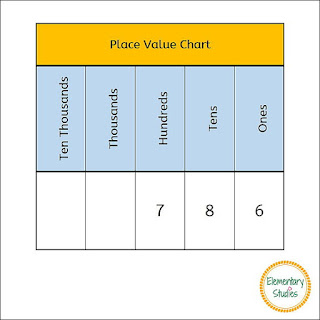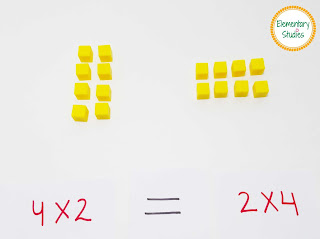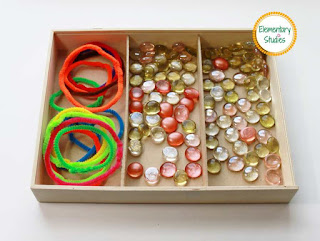## Elementary Studies

### Decimal Place Value

August is already here! Lazy mornings of summer holidays are just flying by and a new school year is starting soon. I will miss the summer fun, but I am more than ready to start another thrilling academic year with new goals, new challenges, and a hope to make this the best school year ever!

While thinking of upper elementary math, the first topic that comes to my mind is a refresher on the number place value system. These young minds already know the whole number place value, but it’s good to refresh the same and introduce the decimal place value too.

The origin of the Arabic number system that we use today can be traced back to the Hindu Scholars in India. This system was adopted and modified by Middle Eastern Scholars and later introduced in Europe. Without going into too much history, let's look at the place value system.

The set of 10 symbols namely 1, 2, 3, 4, 5, 6, 7, 8, 9, 0 represent the numbers.

In the place value system, the position of the number defines the value of the number.

For example, if I write the number 786 then:

• 6 in 786 means 6 ones or 6
• 8 in 786 means 8 tens or 80
• 7 in 786 means 7 hundreds or 700Now for decimals, the place value system extends to the right of ones place to represent the fractions.

For example, if I write the number 786.432 then:
• 4 in 786.432 means 4 tenths or 0.4
• 3 in 786.432 means 3 hundredths or .03
• 2 in 786.432 means 2 thousandths or .002

Check out what’s included in my Decimal Place Value Worksheets and Task Card bundle pack to learn and practice the concept of Place Value for decimals: tenths, hundredths, and thousandths.

Worksheets:
1. Identifying decimals
2. Standard, Expanded and Word Form
3. Decimals on Number Line
4. Comparing decimals
5. Relate Decimals and Fractions
6. Review Sheet
A total of 18 worksheets.

Task-cards to practice the concept of place-value for decimals: tenths, hundredths, and thousandths.
A total of 24 cards along with recording and answer key.

You can grab this Decimal Place Value resource from my store HERE.

Happy to be back in action!

Shimps

### Equivalent Fractions

While teaching equivalent fractions, the very first question that I always ask my students is: What does equivalent means? The answer comes “Equal”.

Yes, Equivalent means Equal in Value or Amount.

Two or more fractions are said to be Equivalent if they represent the same part of a whole.

Example:

These two models are of the same size and both shows the equal shaded parts of a whole. So,
\begin{equation}\frac{1}{3}\textrm{  is equivalent to  }\frac{2}{6}\end{equation}
Another way of showing equivalent fractions is using the number line. Lets look at the same example:

These two red dots are on the same point on the number line and represents the equivalent fraction.
\begin{equation}\frac{1}{3}\textrm{  is equivalent to  }\frac{2}{6}\end{equation}

## Finding Equivalent fractions

Every fraction has many equivalent fractions. You can find these by using multiplication or division.

Multiplication: Multiply the numerator and denominator by the same number. For example:
\begin{equation}\frac{1}{3} \times \frac{2}{2}=\frac{2}{6}\end{equation}
\begin{equation}\frac{1}{3}\textrm{  is equivalent to  }\frac{2}{6}\end{equation}

Division: Divide the numerator and denominator by the same number. For example:
\begin{equation}\frac{5}{10} \div \frac{5}{5}=\frac{1}{2}\end{equation}
\begin{equation}\frac{5}{10}\textrm{  is equivalent to  }\frac{1}{2}\end{equation}
Here is my Equivalent Fractions worksheet and activity pack to introduce, reinforce and practice the concept. This packet is designed primarily for third graders, but can also be used as a review for fourth graders.

This includes the following worksheets and activities on equivalent fractions:
1. Use the fraction area models to complete the number sentence.
2. Use the number line to find the equivalent fractions
3. Find the equivalent fractions without the use of models
4. Equivalent Fractions Ice cream scoop activity
5. Cut and paste activity
6. Make Equivalent Fractions Paper Chain
7. Equivalent Fractions Mystery Pictures

A total of 15 worksheets and activities plus the answer key.

Happy Teaching!

Shimps

### Division Facts

Division is one of the four basic operations of arithmetic, the other being addition, subtraction, and multiplication. Once kids are fluent in multiplication facts, teaching division becomes easy. My today’s post is on introducing the concept of division to the young learners.

#### Introducing Division

Division is a sharing operation, where objects are shared equally into a number of groups.

Hands on Activity: Give 9 pennies or counters and 3 bowls to each child and tell them to put equal pennies/counters in each bowl.

If the result is as shown in picture, tell them - This is what the division is.

#### Multiplication and Division relationship (Multiplication and Division Fact Family)

Multiplication and Division has inverse relationship just like addition and subtraction.

Example:
Multiplication Equation:  4 x 5 = 20
The two division equations will be:
20 ÷ 5 = 4
20 ÷ 4 = 5

Similarly, we can derive multiplication equations from division equation.
35 ÷ 5 = 7

The two multiplication equations will be:
7 x 5 = 35
5 x 7 = 35

Understanding the multiplication and division relationship is very important in solving the division problems.

#### Division Vocabulary

Dividend: A number to be divided by another number.
Divisor: A number by which another number is divided.
Quotient: The result of division.

This division vocabulary poster is also included in my division packet.

You can use this division booklet to teach division facts up to 12 without a remainder. Worksheets covering the above concepts are included in this packet.

This division packet can be purchased from HERE

Happy Teaching!

Shimps

### Properties of Multiplication

In continuation of my posts on the Multiplication topic, today I will be sharing my work on Properties of Multiplication. As per common core standards a 3rd grader is expected to know about these multiplication properties which includes Associative property, Commutative property, Distributive property, Identity property, and Zero property.

This week when I was teaching this topic, I had my kiddo first use the blocks to visualize and see these multiplication properties in action. I am a big fan of these place value blocks and it always comes handy. I bought mine from Lakeshore, but these are now available at Amazon for less 😊. You can get these at Amazon: Place Value Blocks

Later, I gave her the worksheets (HERE) to reinforce her understanding of Properties of Multiplication.

Associative Property of multiplication states that you can change the grouping of the factors the product will be the same.
Example: (5 x 2) x 3 = 30
5 x (2 x 3) = 30

Commutative Property of multiplication states that you can multiply the factors in any order, the product stays the same.
Example: 4 x 2 = 2 x 4Commutative Property of Multiplication

### Multiplication Activities

Hands-on activities are very important in teaching math concepts. Research have shown that students presented with an opportunity to use manipulatives are more interested in mathematics. Today, I am sharing some fun hands-on ways to teach multiplication. Hope you can use and implement some of these ideas in your classroom.

### Multiplication as Equal GroupsMultiplication as Equal Group Activity Box

I bought these pipe cleaners from a Dollar Store. It is super easy to make the hoops with pipe cleaners. Just make a circle and twist at the ends. Ta-da your hoops are ready! For counters, I have used decorative stones which I bought from Michaels (during summer clearance 😊).  You may as well use Buttons, Pom-Poms, M&Ms, Paper Clips, Lego blocks etc.